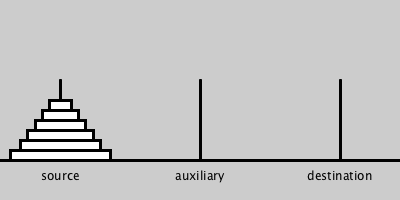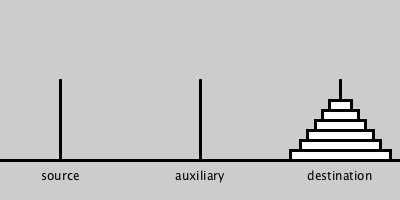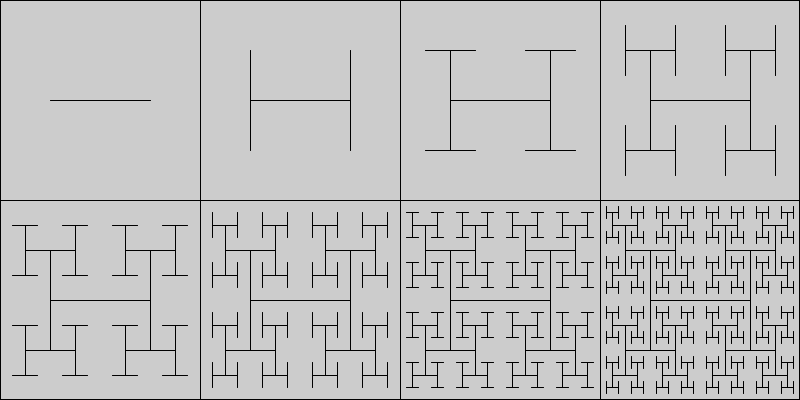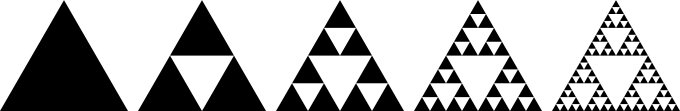# Topics

• Recursive functions
• mutual recursion
• Characteristics of recursive functions
• Tower of Hanoi
• Recursive Graphics
• H-Tree
• Sierpinski triangle
• Tail recursion

# Recursive functions

• The body of a function may contain calls to other functions. In fact, the body of a function f may contain direct calls to f itself.
• A function whose body contains at least a direct call to itself is said to be recursive.
• A function f may be indirectly recursive if it does not call f itself, but one of the functions g that f calls directly calls f (either directly or indirectly).
• When functions f and g are such that f directly calls g and g directly calls f, we say that f and g are mutually recursive.

# Example recursive function

• A canonical example of recursive functions is the factorial function:

fun factorial(n: Int): Int = if (n == 0) 1 else n * factorial(n-1)
• There’s something to be learned even from this simple example.

# Characteristics of recursive functions

• Every recursive function must have base case(s) where the function does not call itself.
• All recursive calls have to end up at the base cases, otherwise the program will get into infinite recursion.
• Implementation of recursion must be done by the runtime system of the programming language.
• This usually involves a stack of activation records. An activation record is a data structure that keeps track of the local variables and other house-keeping information used by an activated function.
• When designing a recursive function, you must not try to follow the recursion. Instead, you must use the magic view of recursion where you assume the function works correctly on smaller cases, and you simply combine the solutions for the smaller cases to make a solution for the larger case.

# Tower of Hanoi

• You are given 3 pegs and n discs of different sizes. All discs are on the source peg, arranged from top to bottom in order of size from smallest to largest.
• You are to find a sequence of moves to put all n discs on the destination peg, using the middle peg as auxillary.
• Each move removes the topmost disc from some peg and put it on another peg subject to the condition that a larger disc may not be put on top of a smaller one.
• The image on the left shows the starting position and and the one on the right shows the ending position.# ToH solution

• This problem can be solved by thinking recursively.
• An optimal solution takes the fewest number of moves possible.
• What is this number as a function of the given number of discs?
• Can you find an optimal solution for every given number of discs?

# ToH solution in Kotlin

• Here is one possible solution to the ToH problem.

/*
* Prints instructions to optimally move n discs from peg src
* to peg des using peg aux as the auxillary peg.
*/
fun hanoi(n: Int, src: Int, aux: Int, des: Int) {
if (n == 1)
println("move top disc from peg $src to peg$des")
else {
hanoi(n-1, src, des, aux)
println("move top disc from peg $src to peg$des")
hanoi(n-1, aux, src, des)
}
}
fun main(args: Array<String>) {
val n = args.toInt()
if (n > 0) hanoi(n, 1, 2, 3)
}
• Exercise: Come up with a more economical solution (shorter code and/or fewer parameters).

# Recursive Graphics

• A recursive graphics is defined in terms of itself.
• A good example is the H-tree, a self-similar fractal tree structure with applications in VLSI design and microwave engineering. The picture below shows the H-trees of level 1 through 8. Can you describe how the level-$$n$$ H-tree is constructed from the level-$$(n-1)$$ H-tree?# Drawing the even-level H-Trees

• This Kotlin code draws the even-level H-Trees.

import processing.core.PApplet

fun main(args: Array<String>) {
PApplet.main("EhtreeSketch");
}

class EhtreeSketch : PApplet() {
var level = 0

override fun settings() {
//size(640, 640)
fullScreen()
noLoop()
}

override fun draw() {
background(250)
ehtree(width/2F, height/2F, width.toFloat(), height.toFloat(), ++level)
level %= 7
}

override fun keyPressed() {
redraw()
}

/*
* Draw a level-2n Htree in the rectangle of width width
* and height height centered at point (x, y).
*/
fun ehtree(x: Float, y: Float, w: Float, h: Float, n: Int) {
if (n < 1) return

val x0 = x - w / 4F
val x1 = x + w / 4F
val y0 = y - h / 4F
val y1 = y + h / 4F

line(x0, y, x1, y)
line(x0, y0, x0, y1)
line(x1, y0, x1, y1)

ehtree(x0, y0, w / 2F, h / 2F, n - 1)
ehtree(x0, y1, w / 2F, h / 2F, n - 1)
ehtree(x1, y0, w / 2F, h / 2F, n - 1)
ehtree(x1, y1, w / 2F, h / 2F, n - 1)
}
}

# Exercise on drawing the H-Trees

• Write a Kotlin program Htree.kt using the processing library such that calling java HtreeKt n will draw a level-n Htree.
• Hint: Imitate the code for drawing the even-level H-Trees and use mutual recursion.

# Sierpinski triangle

• Another example of recursive graphics is the Sierpinski Triangle.
• Here is a picture of the Sierpinski triangle of levels 1, 2, 3, 4, and 5, respectively.• Can you describe how to construct the level-$$n$$ Sierpinski triangle from the level-$$(n-1)$$ Sierpinski triangle?
• Exercise: Write a Kotlin program using the processing library to draw the Sierpinski triangle whose level, side length, and lower left corner are given as parameter. You may assume the triangle is oriented like in the example picture, with its base parallel to the x-axis.

# The cost of recursion

• As mentioned previously, the runtime system of a programming language must provide support for recursive function calls in terms of the stack of activation records.
• A recursive function while executing can potentially be using an enormous amount of memory for the activation records of currently-activated-but-not-yet-finished functions.
• So a recursive function can cause the so-called stack overflow—a condition in which all the stack memory the JVM has allocated for your running program is exhausted, causing your program to abort.
• On the other hand, recursive functions can be a direct, clear, and succinct way to express an algorithm.
• So it would be nice if we can write recursive functions that do not use stack space too heavily.
• It turns out this is possible in some cases.

# Tail recursion

• A recursive function that returns the result of the recursive call as the result for the caller immediately upon completion of the recursive call is said to be tail recursive.
• For example, this version of factorial (to be called with factorial(n, 1) when computing $$n!$$) is tail-recursive

fun factorial(n: Int, acc: Int): Int =
if (n == 0) acc else factorial(n-1, acc*n)
because after the called function factorial(n-1, acc*n) returns, the caller function immediately returns with that result.
• The original version here

fun factorial(n: Int): Int = if (n == 0) 1 else n * factorial(n-1)

is not tail-recursive because after the called function factorial(n-1) returns, the caller function has to multiply that returned result by n before returning with the new result.

# Tail call optimization

• It turns out the Kotlin compiler knows of a way to transform a tail-recursive function call into a loop.
• This is great since loops do not consume stack memory like recursion.
• However, you have to tell the compiler to activate this optimization feature. All you have to do is to put the keyword tailrec in front of the keyword fun at program definition, like this:

tailrec fun factorial(n: Int, acc: Int): Int =
if (n == 0) acc else factorial(n-1, acc*n)